# 2nd PUC Computer Science Model Question Paper 4 with Answers

Students can Download 2nd PUC Computer Science Model Question Paper 4 with Answers, Karnataka 2nd PUC Computer Science Model Question Papers with Answers helps you to revise the complete Karnataka State Board Syllabus and score more marks in your examinations.

## Karnataka 2nd PUC Computer Science Model Question Paper 4 with Answers

Time: 3 Hrs. 15 Min.
Max. Marks: 70

PART-A

I. Each question carries one mark. (10 × 1 =10)

Question 1.
Name the two types of UPS.
The two types of UPS are online UPS and standby UPS.

Question 2.
What are universal gates?
Universal gate is a gate using which ail the basic gates can be designed. NAND and NOR are universal gates.

Question 3.
Give the formula to calculate Base address of one-dimensional array in memory.
The formula to calculate the memory address of an array element is Base_Address + W ( Position – LB ) Where W is number of words per memory cell, position is location of array element for which memory address is calculated, LB is Lower bound (index) of an array.

Question 4.
What do you mean by access specifier ‘private’?
Private access means a member data can only be accessed by the member function of that class only.

Question 5.
What is static memory?
The fixed size of memory allocation and cannot be altered during runtime is called static memory allocation.

Question 6.
What is a field?
Each column in a table is identified by a distinct header is called a field.

Question 7.
What is topology?
It is the geometric arrangement of a computer system in network. Common topologies include a Linear bus, star, ring, and ring.

Question 8.
What is cyber law?
The cyber law has been referred to as the Law of the Internet. Cyberlaw is the part of the overall legal system that deals with the Internet, cyberspace, and their respective legal issues.Question 9.
What is domain affiliation?
A domain affiliation means the type of domain whether it is commercial (.com), education (.edu) or government (.gov), etc.,

Question 10.
What is XML?
XML is a extended Markup Language for documents containing structured information. XML is a text-based markup language becoming the standard for data interchange on the web.

PART – B

II. Each question carries two marks. (5 × 2=10)

Question 11.
Use duality theorem to derive another boolean relation from A + A’B = A+B.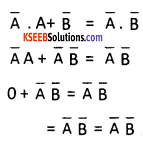Question 12.
Give an example for a Boolean expression the sum of minterms form and product of maxterm form.
The example for boolean expression in the sum of minterms form is X’Y’ + XY’ +XY =Z. The example for Boolean expression in the product of max terms form is x’+y’. x+y’ + x+y =z.

Question 13.
Explain polymorphism.
The polymorphism is ability of an object to take more than one form in different instances. For example, one function name can be used for different purposes. Similarly, same operator can be used for different operations. There are two types one is the “compile-time polymorphism” and other one is the “run-time polymorphism”.

Question 14.
What is a destructor? Which operator is used with destructor?
It is a special member function that destroys the objects that have been created by a constructor when they no longer required. The operator tilde sign (~) is used with destructor.

Question 15.
Name the streams generally used for file I/O.
The streams generally used for file I/O is input stream, output stream, and error stream.

Question 16.
What is data mining?
It is the process of discovering interesting knowledge, such as patterns, associations, changes, anomalies from large amounts of data stored in databases using pattern recognition technologies as well as statistical and mathematical techniques.

Question 17.
How do you modify the column name and width for existing table?
Syntax:
Alter table tablename modify (columnname datatype (size), columnname datatype(size)..);
Example:
Alter table student modify ( studentname varchar2(50) );Question 18.
What is topology? Mention the common topologies.
Topology is the geometric arrangement of a computer system in network. Common topologies include a bus, star, ring and ring topologies.

PART – C

III. Each question carries three marks. (5 × 3 = 15)

Question 19.
Explain Integrated Digital Electronics (IDE) port, Accelerated Graphics Port (AGP) and Small Computer System Interface (SCSI) port.
1. Integrated Digital Eelectronics (IDE) port:
IDE devices like Harddisk drives, CD-ROM drives are connected via 40-pin ribbon cable.

2. Accelerated Graphics Port (AGP):
The AGP is an advanced port designed for Video cards and 3D accelerators designed by Intel. AGP is to provide enhanced graphic accelerator cards, thus enhancing the picture quality of the screen.

3. Small Computer System Interface (SCSI) port:
This port is used for adding external devices such as high-speed hard disks, CD-ROM drives, scanners etc., These ports are expensive but does fast data transfers.

Question 20.
Explain basic gates.
The three basic logic gates are NOT, OR and AND gate.
The NOT or inverter is a gate with only one input and one output. The standards symbol for NOT gate is
The truth table for
1. NOT gate:The truth table for NOT gate:2. OR gate:
The OR gate has two or more input signals but only one output. If any of the input signal is high the output is high.
The standard symbol for OR gate is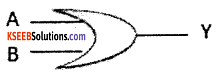The truth table for OR gate.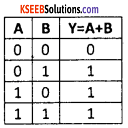3. AND gate:
The AND gate has two or more input signals with one output. When all the inputs are high, then output is high. The standard symbol of AND gate isThe truth table for AND gate:Question 21.
Explain the memory representation of queue using one-dimensional array.
Let Q be a linear array of size N.
The pointer FRONT contains location of front element of the queue (element to be deleted)
The pointer REAR contains location of rear element of the queue (recently added element)
The queue is empty when FRONT = 0
The queue is full when FRONT = N
To add element, REAR = REAR + 1
To delete element, FRONT = FRONT + 1

Question 22.
What is the relationship between string and pointers? Give example.
There is a relationship between string and pointers. In C++ string means character array. When a character array is declared then only its first element address is stored. The rest of the elements can be accessed with the help pointer to character array. This is explained with the following example;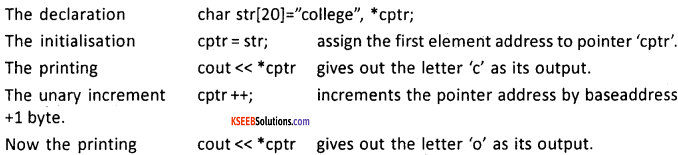Every increment of cptr and printing the value at the location of pointer gives the next element of the character array.Question 23.
Mention the methods of opening files within C++. Discuss anyone.
The methods of opening file within C++ program

• Opening a file using constructor
• Opening a file using member function open() of the class Opening a file using constructor

The.syntax for opening file for output purpose only is ofstream obj(“filename”);
Example:
ofstream out(“results.dat”);

Question 24.
Explain Boyce and Codd form (BCNF).
Boyce and Codd form (BCNF):

• When a relation has more than one candidate key, anomalies may result even though the relation is in 3NF.
• 3NFdoes not deal satisfactorily with the case of relation with overlapping candidate keys
• BCNF is based on the concept of a determinant.
• A determinant is any attribute on which some other attribute is fully functionally dependent.

A relation is in BCNF is, and only if, every determinant is a candidate key.

Question 25.
Write a short note on web servers.
1. A web server is a computer on which a web site is hosted and a program that runs on such a computer. So the term web server refers to both hardware and software.

2. A web site is a collection of web pages stored or “hosted” on a computer that is connected to the internet. Such a computer is known as a Web Server.

3. A web server program is software that runs on the web site hosting Server computer. Its main purpose is serving web pages for requests from web browsers.

4. There are many web server programs available. The most famous and popular of them all is Apache developed by the Apache Foundation. It is free and also available for several operating systems including Windows, Macintosh, and Linux/Unix.

PART D

IV. Each question carries five marks. (7 × 5 = 35)

Question26.
Using maps simplify the expression m1+m3+m5+m6+m7+m9+m11+m13 in four variables W,X,Y,Z.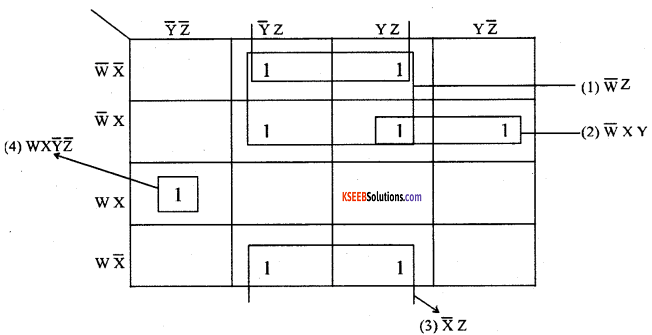Question 27.
Write a short note on linked list.
Linked list is a data structure in which each element is a separate object and each element is dynamically allocated. Each element in the list is called “node”. A linked list is a dynamic data structure. The number of nodes in a list is not fixed and can grow and shrink on demand. Any application which has to deal with an unknown number of objects will need to use a linked list.
The types of linked lists:

1. Singly linked list
2. Doubly linked list
3. Circular linked list

1. A singly linked list:
The node is comprising of two items – the data and a reference to the next node. The last node has a reference to null. The entry point into a linked list is called the head of the list. The head node is reference to the first node. If the list is empty then the head is a null reference.2. A doubly linked list:
is a list that has two references, one to the next node and another to previous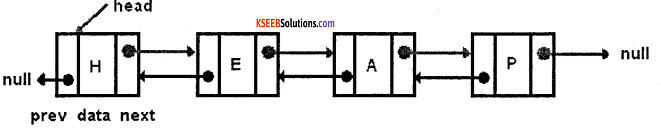3. A circular linked list:
where last node of the list points back to the first node (or the head) of the list.

• Operations on linked lists Creating a linked list
• Traversing a linked list
• Inserting an item into a linked list
• Deleting an item from the linked list
• Searching an item in the linked list
• Merging two or more linked listQuestion 28.
Write an algorithm to insert a data element at the rear end of the queue.
Step 1: if REAR >= N -1 then
Print “Queue is overflow”
Exit
End of if
Step 2: REAR = REAR + 1
Step 3: QUEUE [REAR] = element
Step 4: if FRONT =-1 then
FRONT = 0

Question 29.
Write a short note on concepts of object oriented programming.
Object-oriented programming (OOP) is a programming language model organized around “objects” rather than “actions” and data rather than logic of the program. Object-oriented programming method modularizes a program by creating a memory area for data and member functions together as a single entity.
The following are the basic concepts of object oriented programming.

1. Object:
An object is a combination of code and data that can be treated as a unit. An object can be considered any real time entity (existing thing) that can perform a set of related activities.

2. Class:
The class is a collection of objects that have similar properties and common behavior.

3. Data Abstraction:
The process of hiding the representation of various data items and implementation details of a function from the user program is called data abstraction.

4. Encapsulation:
It is the method of combining the data, attributes, and methods in the same entity is called encapsulation.

Two or more functions with the same name is called function overloading.

6. Polymorphism:
Polymorphism is a Greek word that means Many Shapes. In OOP Polymorphism means the ability to take on many forms. The term is applied both to objects and to functions.

7. Inheritance:
Inheritance is the process by which new objects can acquire the properties of an existing objects of other class.

Question 30.
Explain how objects of a class can be defined?
The objects are declared after a class is defined. The object declaration creates object of that class and memory is allocated for the created object. The object are created using the following
syntax:
classname objectname1, objectname2,
example:
student obj1, obj2;
the obj1 and obj2 are the objects of class type student.
The object names are used to access the data members through member functions by invoking member functions.
example:
obj1.getdata(5, 25);
here, metadata() is the member function of a class student and function call is give using object obj1 which assign the value 5 and 25 to the data members of the class. The memory is allocated separately for data members of each object.Question 31.
Explain friend functions and their characteristics.
A friend function is a non-member function and is a friend of a class. It is declared inside a class with the prefix friend and defined outside the class like any other normal function without the prefix friend. This friend function can access private and protected data members if it is a friend function of that class.

The characteristics of friend function are:

• The friend function should not be defined inside the class
• It can be declared inside a class under public, private or protected access specifier since it is a nonmember function and make no difference.
• A friend function should be defined outside the class without prefix friend
• The scope resolution operator should not be used while defining the friend’s function outside the class since it is not member function of any class.
• It should be called like any other normal function and not be called using an object with dot operator.
• The data members of class are accessed through object and dot operator with data member.

Question 32.
An example show how constructors are used with = operator.
The constructor argument if limited to single argument then = operator can be used to pass the values to these constructors. This is explained in the following example.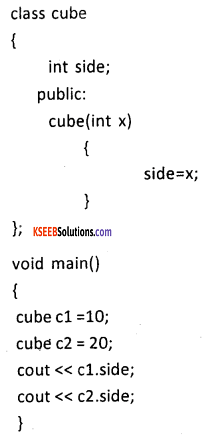Question 33.
Explain single inheritance with a suitable C++program.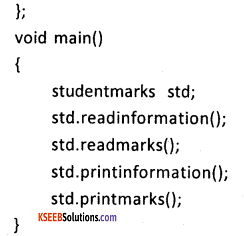Question 40.
Write a short note on Indexed Sequential Access Method.
Indexed Sequential Access Method (ISAM):
This method combines both the feature of sequential and random organization. Records are accessed by using an index or a table. The index stores the address of each record corresponding to a key number of the record.

The records within the file are stored sequentially but directly accessed to individual records.
An indexed sequential access method (ISAM) consists of

1. The main file storage
2. A table to maintain the index

• It combines both sequential and direct
• Suitable for sequential access and random access

• it uses special software and is expensive
• extra time is taken to maintain index
• extra storage for index files
• expensive hardware is required

Question 41.
Classify various built-in functions in SQL.
The built-in functions are classified as Single row functions and Group functions. The single-row functions are of four types. They are numeric functions, character or text functions, date functions, and conversion functions.

1. Numeric functions:
a. ABS function- The ABS function returns the absolute value of the number you point to.

b. CEIL and FLOOR functions – CEIL returns the smallest integer greater than or equal to its argument. FLOOR does just the reverse, returning the largest integer equal to or less than its argument.

c. POWER function – To raise one number to the power of another, use POWER. In this function the first argument is raised to the power of the second.

d. SORT function – The function SORT returns the square root of an argument.

2. Character or text functions:
1. CHR function – returns the character equivalent of the number it uses as an argument.
2. CONCAT function – function combines two strings together.
3. INITCAP function – capitalizes the first letter of a word and makes all other characters lowercase.
4. LOWER and UPPER functions – LOWER changes all the characters to lowercase; UPPER does just the reverse.
5. LENGTH function – returns the length of its lone character argument.

3. Date functions:
1. ADD_MONTHS function – This function adds a number of months to a specified date.
2. LAST_DAY – LAST_DAY function- returns the last day of a specified month.
3. MONTHS_BETWEEN function – to know how many months fall between month x and
month y,
4. NEXT_DAY function – finds the name of the first day of the week that is equal to or later than another specified date.
5. SYSDATE function – SYSDATE returns the system time and date.

4. Conversion functions:

• TO_CHAR function – to convert a number into a character.
• TO_NUMBER function – it converts a string into a number.

The group functions are:

1. AVG() – Returns the average value
2. COUNT() – Returns the number of rows
3. FIRST() – Returns the first value
4. LAST() – Returns the last value
5. MAX() – Returns the largest value
6. MIN() – Returns the smallest value
7. SUM() – Returns the total value of the given columnQuestion 42.
What is gateway? Explain.